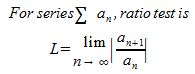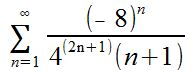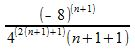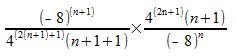# How to Apply the Ratio Test for Convergence & Divergence

Lesson Transcript
Instructor: Yuanxin (Amy) Yang Alcocer

Amy has a master's degree in secondary education and has taught math at a public charter high school.

Watch this video lesson, and you'll learn how you can use the ratio test to help you determine whether a particular series converges or diverges. You will also learn what to watch out for when using the ratio test.

## The Ratio Test

A very useful test that can help you work with series problems is the ratio test. The ratio test helps you to determine whether a particular series converges or diverges. You will need to know this ratio test as you will most likely see this on the standardized tests that you will take as you progress through your schooling and as you prepare for college. Knowing this ratio test will help you to easily answer the problems that ask you whether a certain series converges or not. The ratio test is this:As you can see, to use the test for a particular series an, you take the limit as n approaches infinity of the absolute value of the expression created from the n + 1 term of the series over the n term of the series. Because you are taking the limit of an absolute value, your limit will never be negative.

An error occurred trying to load this video.

Try refreshing the page, or contact customer support.

Coming up next: Testing for Convergence & Divergence by Comparing Series

### You're on a roll. Keep up the good work!

Replay
Your next lesson will play in 10 seconds
• 0:00 The Ratio Test
• 0:52 Rules
• 1:33 Convergence
• 2:57 Divergence
• 3:42 Lesson Summary
Save Save

Want to watch this again later?

Timeline
Autoplay
Autoplay
Speed Speed

## Rules

The convergence and divergence rules for this ratio test are these:

1. If L < 1, then the series converges and is convergent.

2. If L > 1, then the series diverges and is divergent.

3. If L = 1, then the series may be divergent or may be convergent.

For example, if after you take the limit, you get 3 for your answer, then that tells you that your series is divergent because 3 is greater than 1. Now, if you get 10/19 for an answer, then your series is convergent.

Let's take a look at a couple of examples of this ratio test in action now.

## Convergence

First, let's look at this series.The problem asks you to determine whether the series is divergent or convergent. Using the ratio test, you need to write the n + 1 term over the n term and take the limit as n approaches infinity. The n term is the series itself. To find the n + 1 term, all you need to do is to plug in an n + 1 wherever you see an n in the series itself:Even though the ratio test says that you need to place the n + 1 term over the n term, to make it easier for yourself, you can also multiply the n + 1 term by 1 over the n term. Remember that when you divide 1 by a fraction, the fraction inverts where the numerator and denominator switch places.To unlock this lesson you must be a Study.com Member.

### Register to view this lesson

Are you a student or a teacher?